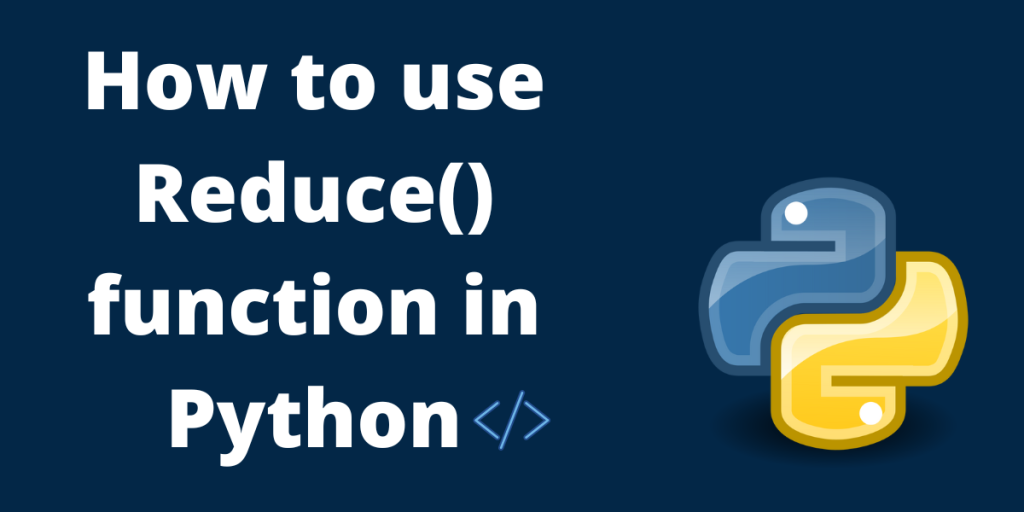# The reduce() function in PythonIn this article, we are going to look at a very important function in python called reduce().

When we write some kind of program, we come across various scenarios when we need to get some kind of mathematical total or an accumulated value to use that result and do something with it.

Let’s say we have an e-commerce website, and we have items and item prices in our shopping cart and we want to calculate the total amount so that a customer can place the order. Well, the reduce() function helps us to calculate that total for us, thus reducing a list of values to a single value.

One more important thing to keep in mind is that the reduce() function doesn’t come as part of the Python built-in functions.  When we download the Python interpreter and the Python package, functools are what we call a tool belt that we can use for functional tools that come with the python installation, and from there we want to import the reduce function as a module.

The functools module is for higher-order functions that act on or return other functions, which in our case provides us with the reduce function.

Let’s go through some code examples to get a better understanding of the reduce() function.

## Implementing Reduce Function in Python

First, we need to import the reduce function from the functools module

```from functools import reduce
```

Declare a list of numbers to perform calculations with it

```my_prices = [40, 50, 60]
```

Let’s define a function for our calculation

```def calc_total(acc, eachItem):
return acc + eachItem

```

Explanation: We are providing two parameters for our function, the acc or the accumulated value, and eachItem, which are used as variables when we will be iterating through our list. Don’t worry, we will come back to the accumulated value to get a better understanding of its use.

Implementing reduce() function:

```total = reduce(calc_total, my_prices, 0)
```

The reduce function takes 3 arguments in total.

1. The first one is the function that we defined earlier in the code.
2. The second one is the list of values or our sequence, that we want to use to perform our operation, also defined previously.
3. The third argument 0, is the initial value accumulated value which technically has a default value of 0, which in our example works fine but in more complicated operations, if we want an initial value of let’s say 1, then it has to be defined as the third argument and the calculation would take 1 as the starting value for the operation.

Explanation: The reduce() function starts with the initial accumulated value (0), takes in our function(calc_total), and uses the list of numbers(my_prices) by iterating over all the items and keeps the accumulated value for each iteration of the performed function and gives back the final result.

Now, that we have our code in place, let’s print out the result

```print(f"The summation for the list is {total}")
```

Output:

``The summation for the list is 150``

## The Complete Code

```# Import reduce
from functools import reduce

# Declare a list
my_prices = [40, 50, 60]

# Define our function
def calc_total(acc, eachItem):
return acc + eachItem

# Use reduce(function_name, data, initial_value)
total = reduce(calc_total, my_prices, 0)

# Print the result
print(f"The summation for the list is {total}")
```

## Conclusion

We see that our code is working as we expected. We are getting the sum as 150. This is how the reduce() function works in Python. It is a very important function in not just Python but in most other programming languages. The basic concepts and use cases for the reduce function are mostly the same in all languages making it worthwhile to get a good understanding of the same.